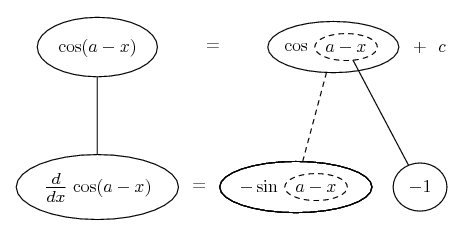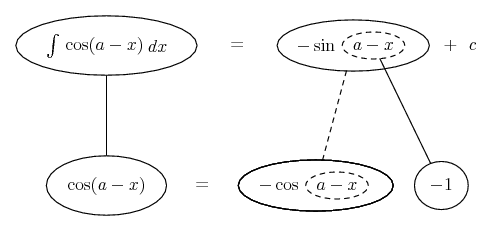# Thread: Int/diff of the form f(x+a) dx

1. ## Int/diff of the form f(x+a) dx

Can anyone tell me the rules concerning integrating or differentiating functions like the following with respect to x

$\displaystyle \int\cos(a-x) dx$

I have only come across those involving x or multiples of x

Thanks

2. See

http://en.wikipedia.org/wiki/Trigonometric_identity

 angle sum and difference identities, also known as the addition and subtraction theorems or formulæ. They were originally established by the 10th century Persian mathematician Abū al-Wafā' al-Būzjānī 

Very interesting!

Kind regards

$\displaystyle \chi$ $\displaystyle \sigma$

3.Originally Posted by bobredCan anyone tell me the rules concerning integrating or differentiating functions like the following with respect to x

$\displaystyle \int\cos(a-x) dx$

I have only come across those involving x or multiples of x

Thanks
You could use the sum formulas chisigma mentions: cos(a- x)= cos(a)cos(x)+ sin(a)sin(x). That gives (cos(a-x))'= -cos(a)sin(x)+ sin(a)cos(x) and $\displaystyle \int cos(a- x)dx= cos(a)sin(x)- sin(a)cos(x)+ C$.

Or you could use the chain rule, for differentiation and a simple substitution of integration ("substitution" is essentially the reverse of the chain rule.). Let u= a- x. Then, by the chain rule $\displaystyle \frac{d cos(a-x)}{dx}= \frac{d cos(u)}{dx}$$\displaystyle = \frac{d cos(u)}{du}\frac{du}{dx}= -sin(u)(-1)= sin(u)= sin(a- x)$.

Or, to integrate $\displaystyle \int cos(a- x)dx$, let u= a- x. Then du= -dx so $\displaystyle \int sin(a- x)dx= -\int cos(u)du= -sin(u)+ C= -sin(a-x)+ C$.

Concerned that the sum formula gives -cos(a)sin(x)+ sin(a)cos(x) for the derivative while the chain rule gives sin(a-x)? Don't be. Use the sum formula for sine again: sin(a-x)= sin(a)cos(-x)+ cos(a)sin(-x)= sin(a)cos(x)- cos(a)sin(x). They are exactly the same thing.

Similarly, -sin(a-x)+ C= -sin(a)cos(-x)- cos(a)sin(-x)+ C= -sin(a)cos(x)+ cos(a)sin(x)+ C. Again, the two methods give the same answer.

4. Just in case a picture helps...

(the chain rule only, not the trigs). Differentiating...... and integrating...As usual, straight continuous lines differentiate downwards, integrate up, the straight dashed line similarly but with respect to the dashed balloon expression.

Don't integrate - balloontegrate!

http://www.ballooncalculus.org/forum/top.php

5. ... use substitution.

Thanks

#### Search Tags

form, int or diff